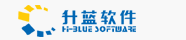# 利用static实现表格的颜色隔行显示

March 25,2004

<?
function getcolor()
{
static \$colorvalue;//定义一个静态变量
if(\$colorvalue=="#ffffff")
\$colorvalue="#000000";
else \$colorvalue="#ffffff";
return(\$colorvalue);
}

print("<table border=1>n");//下面输出10行
for(\$i=0;\$i<10;\$i++)
{ \$bcolor=getcolor();//换背景颜色
print("<tr bgcolor=\$bcolor>n");
print("<td>\$i</td>n");
print("</tr>");
}
print("</table>n");